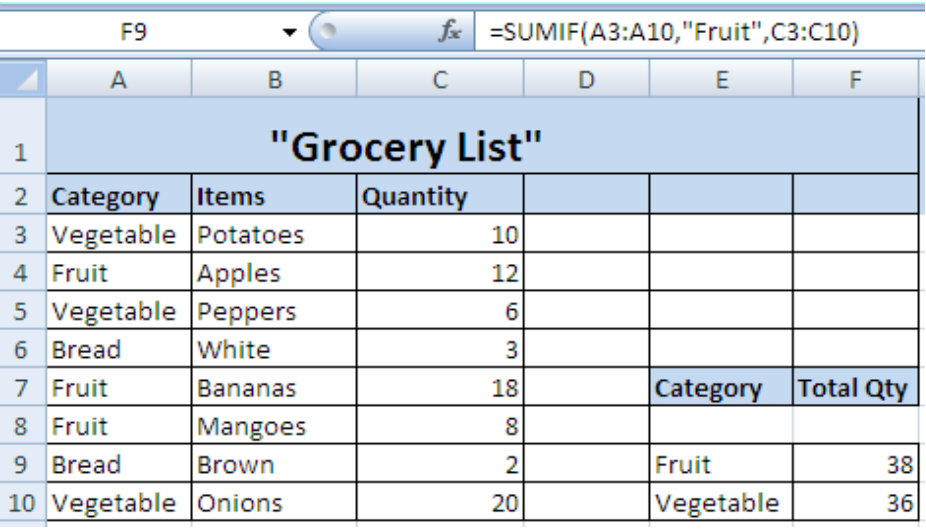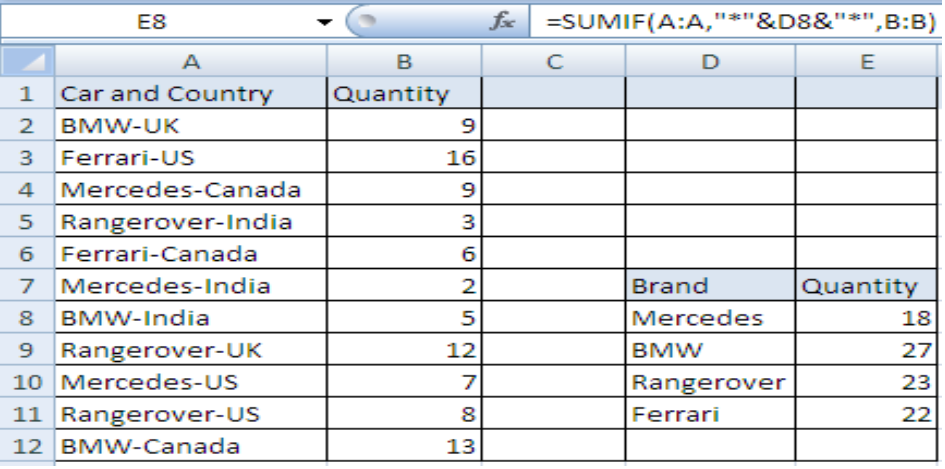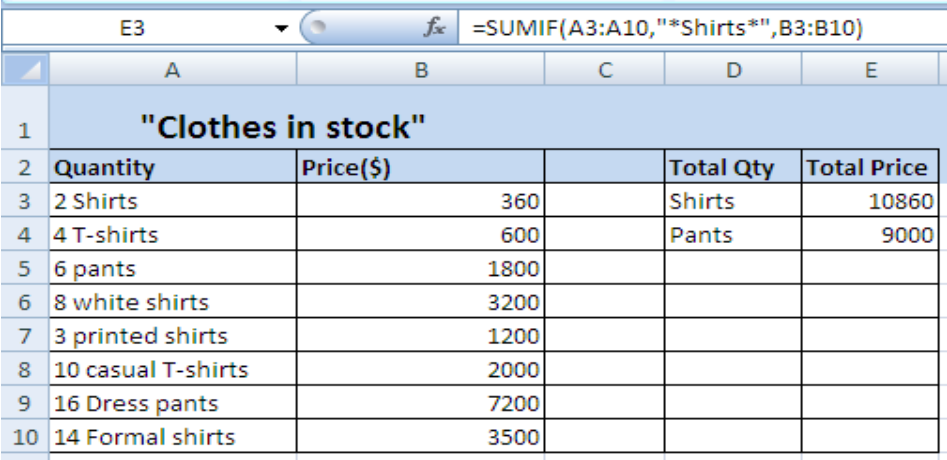Get instant live expert help with Excel or Google Sheets“My Excelchat expert helped me in less than 20 minutes, saving me what would have been 5 hours of work!”

#### Post your problem and you’ll get Expert help in seconds.

Your message must be at least 40 characters
Our professional Expert are available now. Your privacy is guaranteed.

# Using the SUMIF Function to Add Cells if They Contain Certain Text

With the help of SUMIF Function, we can also add cells that contain specific or partial text and even if the cells contain numbers and text altogether, they can also be added.

Let’s have a look on how is it done with a slight change in the formula

## Using SUMIF if cells contain specific textFigure 1. Example 1

Formula for specific text: `=SUMIF(range,”criterianame”,sum_range)`

This is a grocery list with a range that contains specific repeated text. In order to add the sum range based on a specific text criteria, we need to:

• Take a separate column E for the criteria and F for the total quantity.
• Write down the specific criteria in E9 and E10.
• Use SUMIF formula in cell F9 with A3:A10 as range, “Fruit” as criteria instead of E9 and C3:C10 as sum_range.
• Press Enter to get the total quantity of fruit.
• Repeat the same with vegetable criteria to get the amount of total vegetables.

## Using SUMIF if cells contain partial textFigure 2. Example 2

Formula for partial text: `=SUMIF(range,”*”&criteria&”*”,sum_range)`

In this example, we need to use SUMIF function for a partial text in the range as we want to know the total number of cars sold in various countries.

• Take column D for the brand of cars as criteria and column E for the total quantity of cars sold.
• Use SUMIF Formula in E8 with Column A as range and Column B as sum_range whereas criteria D8 will be mentioned with wildcards.
(“*”& on both sides of the criteria D8 is known as wildcards)
• Enter the formula and drag it down to E11 and Excel will automatically calculate the quantities for other criteria’s.

## Using SUMIF if cells contain text and numbersFigure 3. Example 3

Formula for text and numbers: `=SUMIF(range,”*criterianame*”,sum_range)`

We need to find the total price of all the shirts and pants in the given example by taking “Shirts” as one specific criteria with different numbers. Steps to follow are:

• Take column D and write down the specific words “Shirts” and “Pants” that are used with other words and figures in range.
• Take Column E for calculation of total price of all the shirts and pants in range.
• Use formula `=SUMIF(A3:A10,”*Shirts*”,B3:B10) `in E3 and Enter.
• Use the same formula in E4 by only replacing the word “Shirts” with “Pants”.
• We can also use `=SUMIF(A3:A10,"*"&D3&"*",B3:B10)` in E3 and drag it to E4 and we’ll get the same results.

(The second formula given in this example is the same one that is used in example # 2.It can be used in both cases)

Most of the time, the problem you will need to solve will be more complex than a simple application of a formula or function. If you want to save hours of research and frustration, try our live Excelchat service! Our Excel Experts are available 24/7 to answer any Excel question you may have. We guarantee a connection within 30 seconds and a customized solution within 20 minutes.

Solution examplesamending a formula so that there is another criteria and if it does not meet this criteria then we stick with the original criteria
Solved by A. B. in 40 minsI need Column D to = Column C - Column A only if Column B has a 'Date' entered.
Solved by I. B. in 11 minsHello, I need help trying to create a sum of numerical values on a spreadsheet in which the only values that apply to the sum are when the status of another cell is a certain value (e.g. value is not in sum when status: closed, value is in sum when status: open)
Solved by T. W. in 18 minsI am looking for a formula to SUM column L, if Column A matched the relevant date and column C matches the name
Solved by Z. Y. in 16 minsI need a formula that is essentially saying IF this range of cells contain a certain name, add the number in another cell on the same row, and them sum that number in a different cell..!
Solved by O. H. in 44 mins